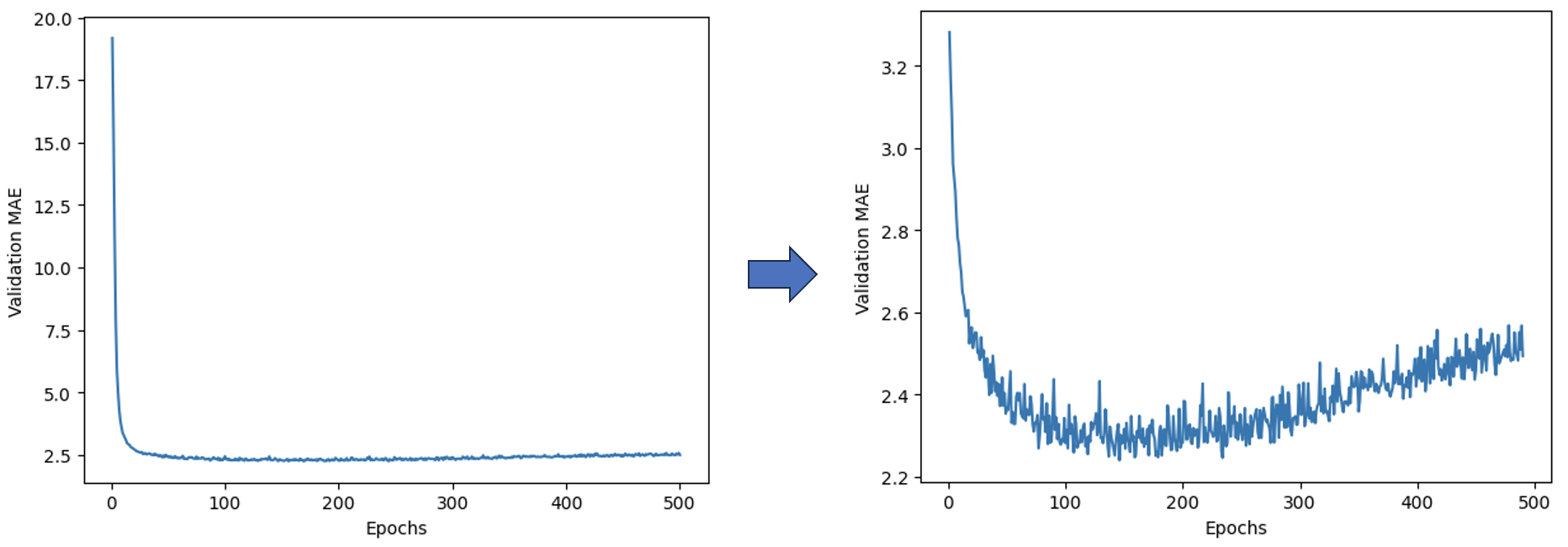## 2. Keras和TensorFlow入门

TensorFlow是基于Python的开源机器学习框架，目前的最新稳定版本是v2.12.0。注意，TensorFlow v2.x版本的API与v1.x版本的有很多不同之处。Keras则是构建于TensorFlow上的开源机器学习API库。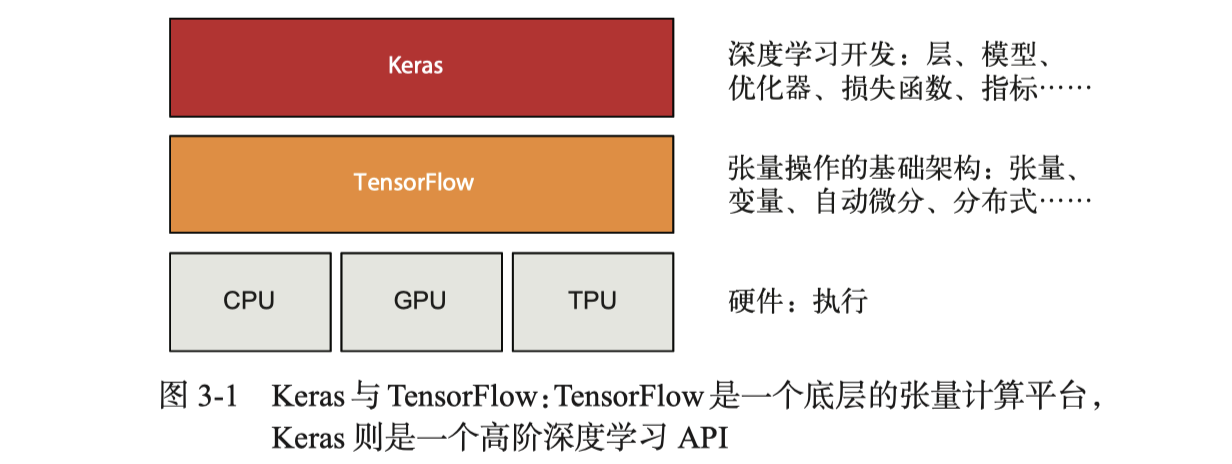``````>>> tf.ones(shape=(2, 1))
<tf.Tensor: shape=(2, 1), dtype=float32, numpy=
array([[1.],
[1.]], dtype=float32)>
>>> tf.zeros(shape=(2, 1))
<tf.Tensor: shape=(2, 1), dtype=float32, numpy=
array([[0.],
[0.]], dtype=float32)>
>>> tf.random.normal(shape=(3, 1), mean=0., stddev=1.)
<tf.Tensor: shape=(3, 1), dtype=float32, numpy=
array([[1.2883002],
[1.359465 ],
[1.1576971]], dtype=float32)>
``````

``````>>> x = np.ones(shape=(2, 2))
>>> x[0, 0] = 0.
>>> y = tf.ones(shape=(2, 2))
>>> y[0, 0] = 0.
Traceback (most recent call last):
File "<stdin>", line 1, in <module>
TypeError: 'tensorflow.python.framework.ops.EagerTensor' object does not support item assignment
>>> v = tf.Variable(initial_value=tf.random.normal(shape=(3, 1)))
>>> v[0, 0].assign(3.)
<tf.Variable 'UnreadVariable' shape=(3, 1) dtype=float32, numpy=
array([[ 3.        ],
[-0.6722511 ],
[-0.09299621]], dtype=float32)>
``````

`tf.square`用于求平方，`tf.sqrt`用于求平方根，星号用于两个张量逐元素的积运算，`tf.matmul`则是点积运算。这些简单运算都是急切执行（eager execution）的运算。从TensorFlow 2.0起，急切执行默认开启。

### 2.1 用TensorFlow编写线性分类器

``````# generate data
num_samples_per_class = 1000
negative_samples = np.random.multivariate_normal(mean=[0, 3], cov=[[1, 0.5], [0.5, 1]], size=num_samples_per_class)
positive_samples = np.random.multivariate_normal(mean=[3, 0], cov=[[1, 0.5], [0.5, 1]], size=num_samples_per_class)
# generate input data
inputs = np.vstack((negative_samples, positive_samples)).astype(np.float32)
# generate labels
targets = np.vstack((np.zeros((num_samples_per_class, 1), dtype="float32"), np.ones((num_samples_per_class, 1), dtype="float32")))
``````

``````plt.scatter(inputs[:, 0], inputs[:, 1], c=targets[:, 0])
plt.show()
``````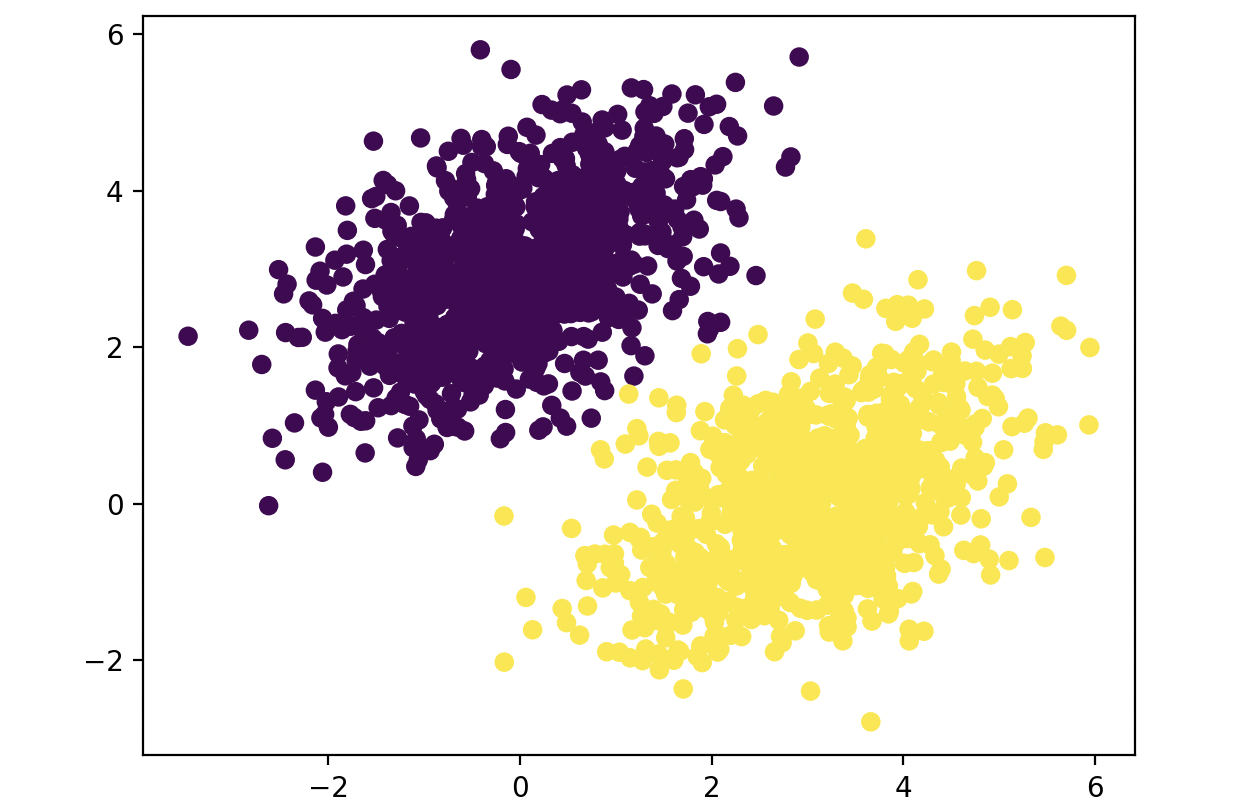``````input_dim = 2
output_dim = 1
W = tf.Variable(initial_value=tf.random.uniform(shape=(input_dim, output_dim)))
b = tf.Variable(initial_value=tf.zeros(shape=(output_dim,)))

def model(inputs): # forward propagation
return tf.matmul(inputs, W) + b

def square_loss(targets, predictions): # loss function
per_sample_losses = tf.square(targets - predictions)
return tf.reduce_mean(per_sample_losses)
``````

``````# training step
learning_rate = 0.1

def training_step(inputs, targets):
predictions = model(inputs)
loss = square_loss(targets, predictions)
return loss

for step in range(40): # training
loss = training_step(inputs, targets)
print(f"Loss at step {step}: {loss:.4f}")
``````

40轮迭代结束后，我们看一下模型的分类能力如何：

``````# visualization
predictions = model(inputs)
x = np.linspace(-1, 4, 100)
y = - W / W * x + (0.5 - b) / W
plt.plot(x, y, "-r")
plt.scatter(inputs[:, 0], inputs[:, 1], c=predictions[:, 0] > 0.5)
plt.show()
``````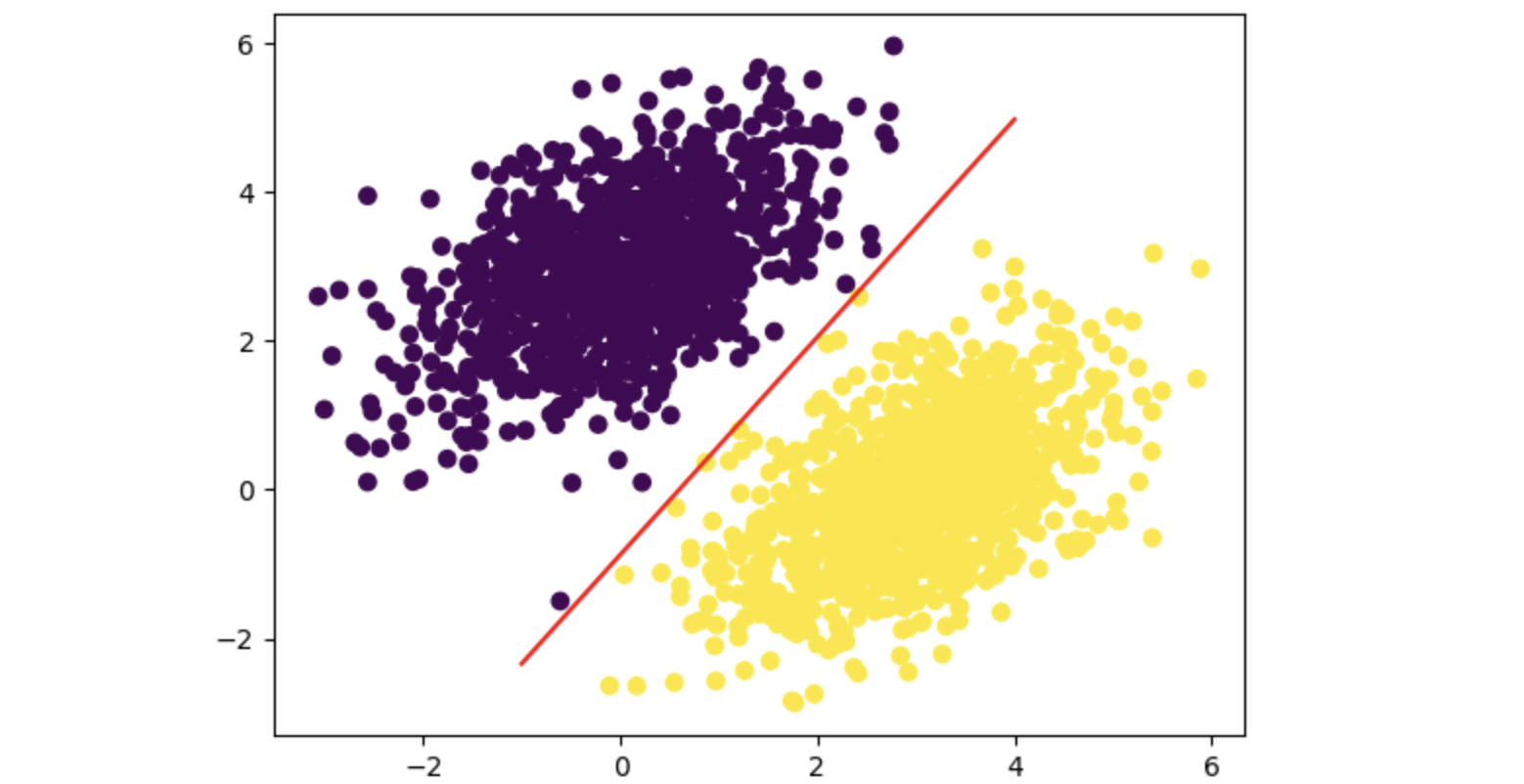### 2.2 了解核心Keras API

#### 2.2.1 层：深度学习的基础模块

Keras的核心概念是Layer类，它是封装了状态（权重，在`build()`方法中定义或在构造函数中创建）和计算（一次前向传播，在`call()`方法中定义）的对象。Keras中的其他东西要么是Layer，要么与Layer密切交互。我们可以基于它设计自己的层。下面是一个全连接层的实现：

``````class SimpleDense(keras.layers.Layer):
def __init__(self, units, activation=None):
super().__init__()
self.units = units
self.activation = activation

def build(self, input_shape):
input_dim = input_shape[-1]
initializer="random_normal")
initializer="zeros")

def call(self, inputs):
y = tf.matmul(inputs, self.W) + self.b
if self.activation is not None:
y = self.activation(y)
return y
``````

``````my_dense = SimpleDense(units=32, activation=tf.nn.relu)
input_tensor = tf.ones(shape=(2, 784))
output_tensor = my_dense(input_tensor)
``````

``````from tensorflow.keras import models
model = keras.Sequential([
SimpleDense(32, activation="relu"),
SimpleDense(64, activation="relu"),
SimpleDense(32, activation="relu"),
SimpleDense(10, activation="softmax")
])
``````

``````def __call__(self, inputs):
if not self.built:
self.build(inputs.shape)
self.built = True
return self.call(inputs)
``````

#### 2.2.3 编译步骤：配置学习过程

``````model = keras.Sequential([
layers.Dense(512, activation="relu"),
layers.Dense(10, activation="softmax")
])
model.compile(optimizer="rmsprop",
loss="sparse_categorical_crossentropy",
metrics=["accuracy"])
``````

``````optimizer=keras.optimizers.RMSprop(learning_rate=1e-4)
``````

#### 2.2.5 理解`fit()`方法

`fit()`方法将开始执行训练循环，接收的参数有：训练数据（输入和目标）、训练轮数、批量大小等：

``````history = model.fit(inputs, targets, epochs=5, batch_size=128)
``````

#### 2.2.6 监控验证数据上的损失和指标

``````indices_permutation = np.random.permutation(len(inputs))
shuffled_inputs = inputs[indices_permutation]
shuffled_targets = targets[indices_permutation]
num_validation_samples = int(0.3 * len(inputs))
val_inputs = shuffled_inputs[:num_validation_samples]
val_targets = shuffled_targets[:num_validation_samples]
training_inputs = shuffled_inputs[num_validation_samples:]
training_targets = shuffled_targets[num_validation_samples:]
model.fit(
training_inputs,
training_targets,
epochs=5,
batch_size=16,
validation_data=(val_inputs, val_targets)
)
``````

``````loss_and_metrics = model.evaluate(val_inputs, val_targets, batch_size=128)
``````

#### 2.2.7 推断：在训练后使用模型

``````predictions = model.predict(val_inputs, batch_size=128)
``````

## 3. 神经网络入门：分类与回归

### 3.1 影评分类：二分类问题示例

#### 3.1.1 IMDB数据集

``````from tensorflow.keras.datasets import imdb
(train_data, train_labels), (test_data, test_labels) = imdb.load_data(num_words=10000)
``````

Keras中内置的IMDB数据集已经经过预处理——单词序列被转换为整数序列，每个整数对应字典中的某个单词。`num_words`用来指定仅保留训练数据中前10000个最常出现的单词，从而避免向量数据过大。

``````word_index = imdb.get_word_index()
reverse_word_index = dict([(value, key) for (key, value) in word_index.items()])
decoded_review = " ".join([reverse_word_index.get(i - 3, "?") for i in train_data])
``````

#### 3.1.2 准备数据

1. 填充列表，使长度相等，再将列表转换为`(samples, max_length)`形状的整数张量。模型第一层使用Embedding层来处理这种张量。
2. 对列表进行multi-hot编码，转换成由0和1组成的10000维向量，原整数序列中的元素指示该向量中对应索引处为1，其余为0。模型第一层使用Dense层。

``````def vectorize_sequences(sequences, dimension=10000):
results = np.zeros((len(sequences), dimension))
for i, sequence in enumerate(sequences):
for j in sequence:
results[i, j] = 1.
return results
x_train = vectorize_sequences(train_data)
x_test = vectorize_sequences(test_data)
y_train = np.asarray(train_labels).astype("float32")
y_test = np.asarray(test_labels).astype("float32")
``````

``````>>> print(x_train.shape, x_test.shape, y_train.shape, y_test.shape)
(25000, 10000) (25000, 10000) (25000,) (25000,)
``````

#### 3.1.3 构建模型

``````model = keras.Sequential([
layers.Dense(16, activation="relu"),
layers.Dense(16, activation="relu"),
layers.Dense(1, activation="sigmoid")
])
``````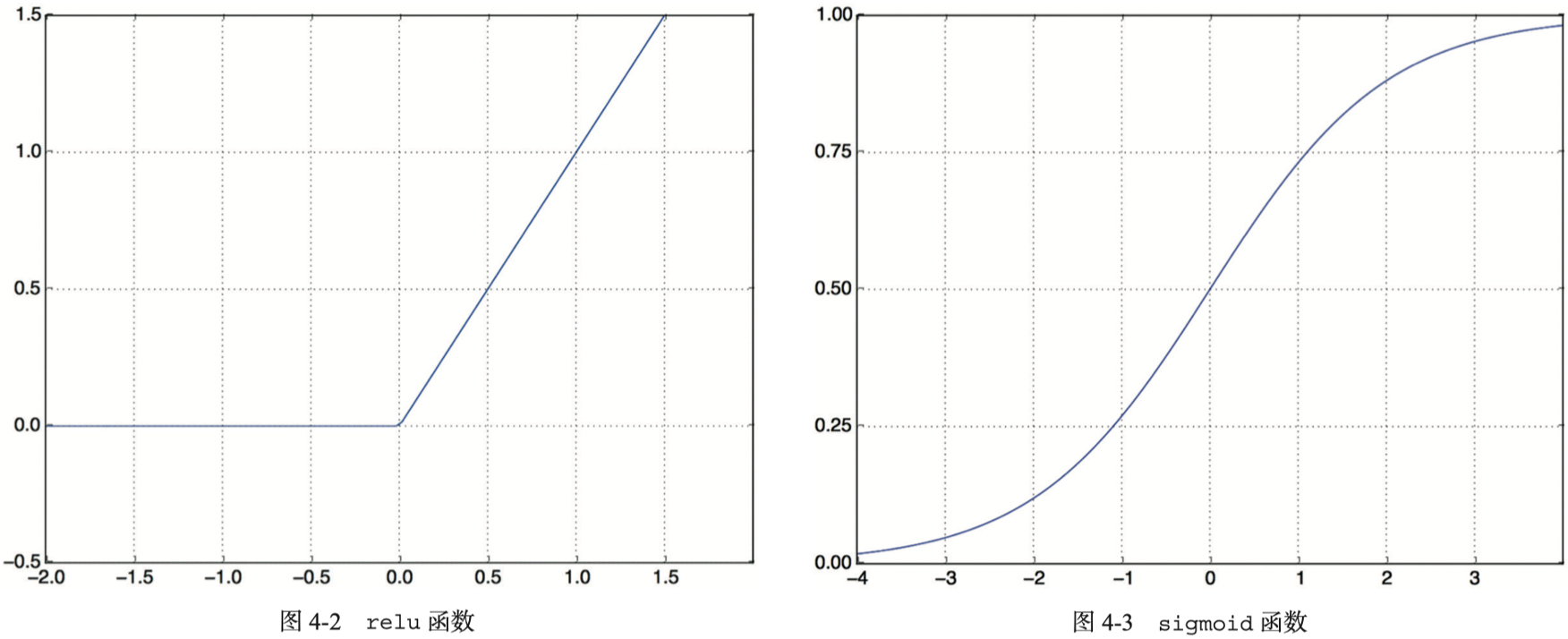``````model.compile(optimizer="rmsprop", loss="binary_crossentropy", metrics=["accuracy"])
``````

#### 3.1.4 验证方法

``````x_val = x_train[:10000]
partial_x_train = x_train[10000:]
y_val = y_train[:10000]
partial_y_train = y_train[10000:]

history = model.fit(partial_x_train,
partial_y_train,
epochs=20,
batch_size=512,
validation_data=(x_val, y_val))
``````

``````import matplotlib.pyplot as plt
history_dict = history.history
loss_values = history_dict["loss"]
val_loss_values = history_dict["val_loss"]
epochs = range(1, len(loss_values) + 1)
plt.plot(epochs, loss_values, "bo", label="Training loss")
plt.plot(epochs, val_loss_values, "b", label="Validation loss")
plt.title("Training and validation loss")
plt.xlabel("Epochs")
plt.ylabel("Loss")
plt.legend()
plt.show()

plt.clf()
acc = history_dict["accuracy"]
val_acc = history_dict["val_accuracy"]
plt.plot(epochs, acc, "bo", label="Training acc")
plt.plot(epochs, val_acc, "b", label="Validation acc")
plt.title("Training and validation accuracy")
plt.xlabel("Epochs")
plt.ylabel("Accuracy")
plt.legend()
plt.show()
``````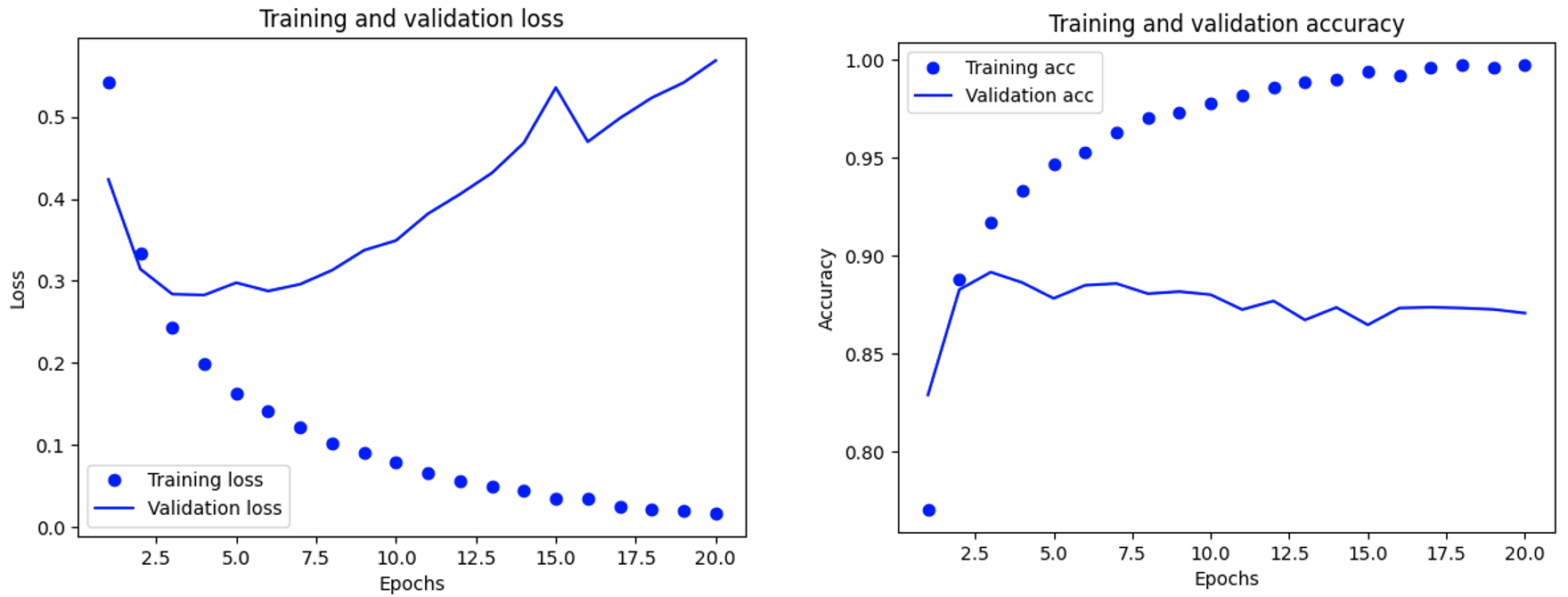``````model.fit(x_train, y_train, epochs=4, batch_size=512)
results = model.evaluate(x_test, y_test)
# results: [0.28500404953956604, 0.885919988155365]
``````

``````model.predict(x_test)
``````

### 3.2 新闻分类：多分类问题示例

#### 3.2.1 路透社数据集

``````from tensorflow.keras.datasets import reuters
(train_data, train_labels), (test_data, test_labels) = reuters.load_data(num_words=10000)
``````

#### 3.2.2 准备数据

``````x_train = vectorize_sequences(train_data)
x_test = vectorize_sequences(test_data)
``````

1. 将标签列表转换为一个整数张量。
2. One-hot编码（也叫分类编码，categorical encoding），与multi-hot类似，将每个标签表示为全零向量，只有标签索引位置的元素为1。

``````def to_one_hot(labels, dimension=46):
results = np.zeros((len(labels), dimension))
for i, label in enumerate(labels):
results[i, label] = 1.
return results
y_train = to_one_hot(train_labels)
y_test = to_one_hot(test_labels)
``````

``````from tensorflow.keras.utils import to_categorical
y_train = to_categorical(train_labels)
y_test = to_categorical(test_labels)
``````

#### 3.2.3 构建模型

``````model = keras.Sequential([
layers.Dense(64, activation="relu"),
layers.Dense(64, activation="relu"),
layers.Dense(46, activation="softmax")
])
``````

``````model.compile(optimizer="rmsprop", loss="categorical_crossentropy", metrics=["accuracy"])
``````

#### 3.2.4 验证方法

``````history = model.fit(partial_x_train,
partial_y_train,
epochs=20,
batch_size=512,
validation_data=(x_val, y_val))
``````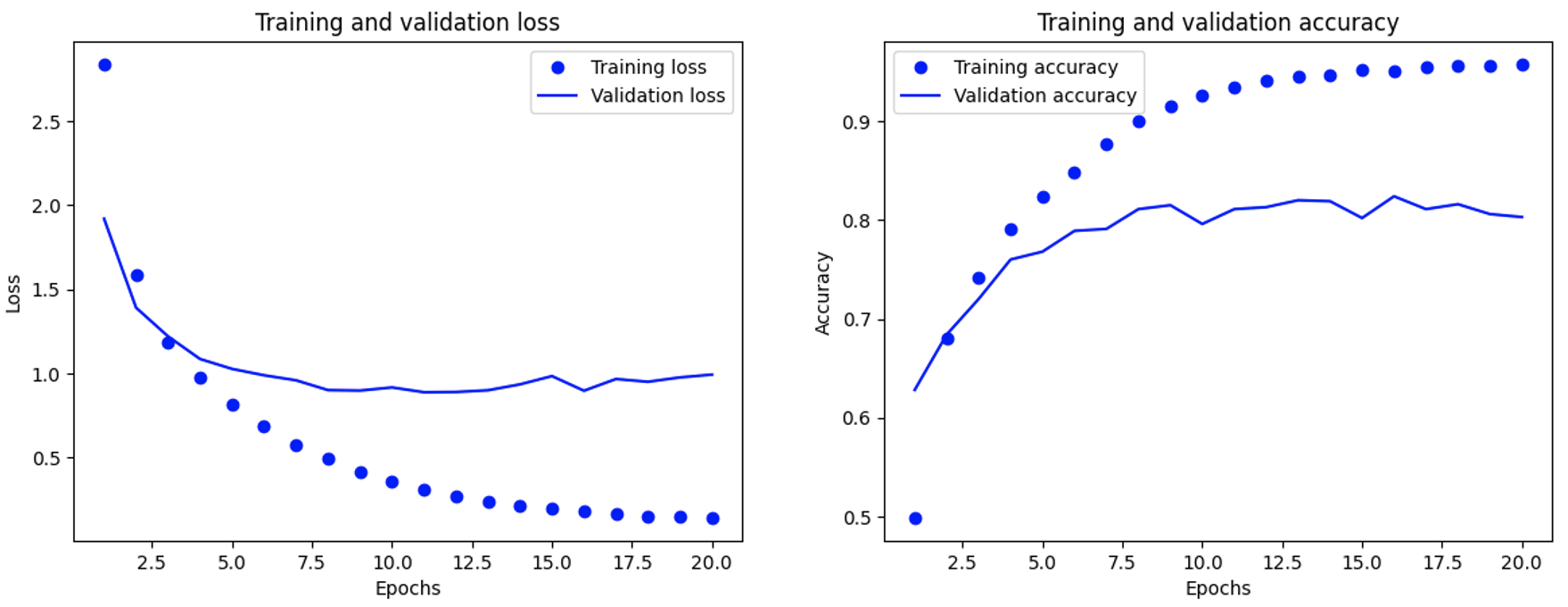``````>>> predictions = model.predict(x_test)
>>> np.argmax(predictions)
3
``````

#### 3.2.5 处理标签和损失的另一种方法

``````y_train = np.array(train_labels)
y_test = np.array(test_labels)
model.compile(optimizer="rmsprop", loss="sparse_categorical_crossentropy", metrics=["accuracy"])
``````

#### 3.2.6 拥有足够大的中间层的重要性

``````model = keras.Sequential([
layers.Dense(64, activation="relu"),
layers.Dense(4, activation="relu"),
layers.Dense(46, activation="softmax")
])
``````

### 3.3 房价预测：标量回归问题示例

#### 3.3.1 波士顿房价数据集

``````from tensorflow.keras.datasets import boston_housing
(train_data, train_targets), (test_data, test_targets) = boston_housing.load_data()
``````

``````>>> train_data.shape
(404, 13)
>>> test_data.shape
(102, 13)
``````

#### 3.3.2 准备数据

``````mean = train_data.mean(axis=0)
train_data -= mean
std = train_data.std(axis=0)
train_data /= std
test_data -= mean
test_data /= std
``````

#### 3.3.3 构建模型

``````def build_model():
model = keras.Sequential([
layers.Dense(64, activation="relu"),
layers.Dense(64, activation="relu"),
layers.Dense(1)
])
model.compile(optimizer="rmsprop", loss="mse", metrics=["mae"])
return model
``````

#### 3.3.4 利用K折交叉验证来验证方法``````k = 4
num_val_samples = len(train_data) // k
num_epochs = 100
all_scores = []
for i in range(k):
print(f"Processing fold #{i}")
val_data = train_data[i * num_val_samples: (i + 1) * num_val_samples]
val_targets = train_targets[i * num_val_samples: (i + 1) * num_val_samples]
partial_train_data = np.concatenate(
[train_data[:i * num_val_samples],
train_data[(i + 1) * num_val_samples:]],
axis=0)
partial_train_targets = np.concatenate(
[train_targets[:i * num_val_samples],
train_targets[(i + 1) * num_val_samples:]],
axis=0)
model = build_model()
model.fit(partial_train_data, partial_train_targets,
epochs=num_epochs, batch_size=16, verbose=0)
val_mse, val_mae = model.evaluate(val_data, val_targets, verbose=0)
all_scores.append(val_mae)
``````

``````>>> all_scores
[2.045789957046509, 2.4252355098724365, 2.456112861633301, 2.522847890853882]
>>> np.mean(all_scores)
2.362496554851532
``````

``````num_epochs = 500
all_mae_histories = []
for i in range(k):
# ...
history = model.fit(partial_train_data, partial_train_targets, validation_data=(val_data, val_targets), epochs=num_epochs, batch_size=16, verbose=0)
mae_history = history.history["val_mae"]
all_mae_histories.append(mae_history)

# 计算每轮训练的K折验证分数平均值
average_mae_history = [np.mean([x[i] for x in all_mae_histories]) for i in range(num_epochs)]
``````

``````# for left subgraph below
plt.plot(range(1, len(average_mae_history) + 1), average_mae_history)
plt.xlabel("Epochs")
plt.ylabel("Validation MAE")
plt.show()

# for right subgraph below
truncated_mae_history = average_mae_history[10:]
plt.plot(range(1, len(truncated_mae_history) + 1), truncated_mae_history)
plt.xlabel("Epochs")
plt.ylabel("Validation MAE")
plt.show()
``````